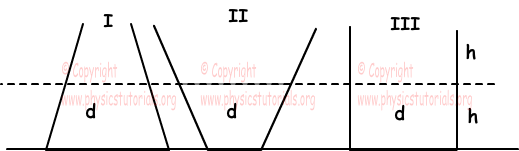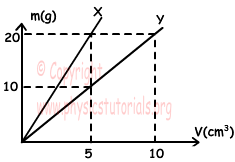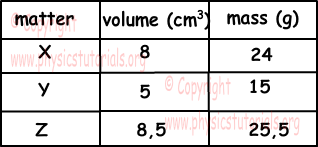Properties of Matter Exam2 and Problem Solutions

Properties of Matter Exam2 and  Problem Solutions

1. There are three different tanks filled with water. If we fill rest of the tanks with liquid having density 0,8g/cm3, find the relation between the final densities of homogeneous mixtures.In the first tank, volume of water is larger than the volume of added liquid. Thus, density of mixture1 is closer to the density of water.

In the second tank, volume of water is smaller than the volume of added liquid. Thus, density of mixture2 is closer to the density of added liquid.

In the third tank, volume of water is equal to the volume of added liquid. Thus, density of mixture3 is ;

dmixture3=(1+0,8)/2=0,9g/cm3

Relation between densities of mixtures;

d1>d3>d2

2. An object made of matter having density 6g/cm3 has mass 1500g. Find the volume of space inside the object.

m=V.d

1500=V.6

v=150cm3

Object has volume 600 cm3, thus volume of the space is;

600-250=350cm3.

3. Mass vs. volume graph of matters X and Y is given below. Find the density of mixtures;

a) If we take equal amount of mass from X and Y

b) If we take equal amount of volume from X and Ya) dmixture1=(mx+my)/(Vx+Vy)=(20+20)/(5+10)=8/3g/cm3

b) dmixture1=(mx+my)/(Vx+Vy)=(10+20)/(5+5)=3g/cm3

4. Table given below shows the properties of three matters. Find whether these matters are same or not.If the densities of these matters X, Y and Z are equal at same temperature and pressure, we can say they are same matters.

dX=mX/Vx=24/8=3g/cm3

dY=mY/VY=15/5=3g/cm3

dZ=mZ/VZ=25,5/8,5=3g/cm3

X, Y and Z can be same matter.

5. Which one/ones of the following properties is/are distinguishing property of matter.

a)Volume

b) Mass

c)Density

d) Elasticity

e) Melting point

Volume and mass are common properties of matters. Density is distinguishing property of solid, liquid and gas  form of matters. On the contrary, elasticity and melting point are distinguishing properties of solid matters.

Author:

Tags: